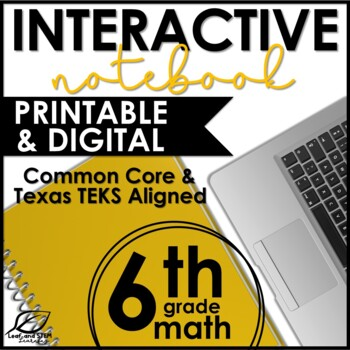# Sixth Grade Math Interactive Notebook GROWING BundleSubject
Resource Type
File Type

Zip

(63 MB|260+)
Standards
13 Products in this Bundle
13 products
Bonus
1 file
Standards Alignment Document
• Bundle Description
• StandardsNEW

A bundle of low prep, highly engaging, and academically relevant interactive math notebook materials for your 6th grade curriculum? Yes... here it is! This GROWING bundle is a huge value for any Common Core or Texas TEKS sixth grade math classroom.

How is this bundle different than others available?

•Each notebook set has three parts: a half page notes, interactive and often reusable practice activities, and a test prep style assessment. Most other notebook bundles offer only a set of notes.

•Includes word problems and models for every included topic. This set is about deep learning, not just cutesy notes.

•Easy and efficient to use. The notes waste no space for teachers who have limited copies and paper. Each activity requires only a few scissor cuts for students.

•Developed with guided math and differentiation in mind! Buyers have used these materials with multiple levels of students successfully, from pre ap classes to ESL classes; as an extension for gifted students and as a support for those with special needs.

This is a Growing Bundle--this means that more sets will be added in the future. You are paying only for the sets that are currently included, but you will be able to download all future sets added for no addition cost! Each time a new notebook set is added, the price will increase until the bundle is complete. Buy now to lock in the lowest available price. The preview is a clickable and downloadable file with the Common Core and TEKS alignment guide. It also has information on planned sets to be added in the future. Check it out!

Topics Currently Available:

Rational Number System Interactive Notebook Set

• The Rational Number System

• Whole Numbers

• Integers

• Rational Numbers

• Comparing Rational Numbers

• Ordering Rational Numbers

• Absolute Value

• Opposite Numbers

• Fraction Notation of Division

Positive Rational Number Operations Interactive Notebook Set

• Mixed Additive Positive Rational Number Operations

• Multiplying Decimals Models

• Multiplying Decimals using the Algorithm

• Dividing Decimals with Models

• Dividing Decimals using the Algorithm

• Multiplying Fractions with Models
• Multiplying Fractions using the Algorithm
• Dividing Fractions with Models
• Finding Reciprocals
• Dividing Fractions using the Algorithm

Order of Operations Interactive Notebook Set

• Order of Operations

• Levels of Grouping

• Simplifying using Distribution

• Order of Operations with Integers

Prime Factorization Interactive Notebook Set

• What is an Exponent?

• Prime Factorization

• Prime Factor Trees

• Division by Primes (Cake)

Properties of Operations Interactive Notebook Set

• Associative Property

• Commutative Property

• Distributive Property

• Inverse Property

• Identity Property

Integer Interactive Notebook Set- Vocabulary and Integer Operations

• Money Model Integer Vocabulary

• Elevation Model Integer Vocabulary

• Temperature Model Integer Vocabulary

• Writing Integers

• Combining Integers

• Grouping Integers

• Multiplying and Dividing Integers

• Integer Operation Quick Reference

One-Step Equations Interactive Notebook Set

• Introduction to Equations

• Modeling Equations with Objects

• Modeling Equations with Algebra Tiles

• Multiplicative Equations

• Fraction Equations

• Integer Equations

Ratios and Rates Interactive Notebook Set

• Fraction and Decimals

• Decimals and Percents

• Fractions and Percents

• Fraction Decimal Percent Mnemonic

• Fraction and Percent Strip Diagrams

• What is a Ratio?

• Percentages as a Ratio

• Scaling Ratios

• What is a Rate?

• Unit Rates

Proportionality Interactive Notebook Set

• Introduction to Proportionality

• Ratio Tables

• Graphing Proportionality

• Tape Diagrams for Proportionality

• Qualitative Proportionality

• Quantitative Proportionality (Proportion Equations)

Area and Volume Interactive Notebook Set

• Decomposing Parallelograms

• Decomposing Triangles

• Decomposing Trapezoids

• Area of Parallelograms

• Area of Triangles

• Area of Trapezoids

• Area of Composite Figures

• Volume of Rectangular Prisms

Data and Statistics Interactive Notebook Set

•What is Variability?

•Dot Plots

•Stem-and-Leaf Plots

•Histograms

•Box Plots

•Measures of Central Tendency

•Mode

•Median

•Mean

•Range

•Interquartile Range (IQR)

•Mean Absolute Deviation (for CCSS classrooms)

•Skew and Symmetry

•Gaps, Clusters, and Outliers

Are you a Texas teacher? These notebooks are also a part of the 6th Grade Math TEKS Mega Bundle!

Solve unit rate problems including those involving unit pricing and constant speed. For example, if it took 7 hours to mow 4 lawns, then at that rate, how many lawns could be mowed in 35 hours? At what rate were lawns being mowed?
Make tables of equivalent ratios relating quantities with whole-number measurements, find missing values in the tables, and plot the pairs of values on the coordinate plane. Use tables to compare ratios.
Use ratio and rate reasoning to solve real-world and mathematical problems, e.g., by reasoning about tables of equivalent ratios, tape diagrams, double number line diagrams, or equations.
Understand the concept of a unit rate 𝘢/𝘣 associated with a ratio 𝘢:𝘣 with 𝘣 ≠ 0, and use rate language in the context of a ratio relationship. For example, “This recipe has a ratio of 3 cups of flour to 4 cups of sugar, so there is 3/4 cup of flour for each cup of sugar.” “We paid \$75 for 15 hamburgers, which is a rate of \$5 per hamburger.”
Understand the concept of a ratio and use ratio language to describe a ratio relationship between two quantities. For example, “The ratio of wings to beaks in the bird house at the zoo was 2:1, because for every 2 wings there was 1 beak.” “For every vote candidate A received, candidate C received nearly three votes.”
Total Pages
260+
Included
Teaching Duration
1 Semester
Report this Resource to TpT
Reported resources will be reviewed by our team. Report this resource to let us know if this resource violates TpT’s content guidelines.